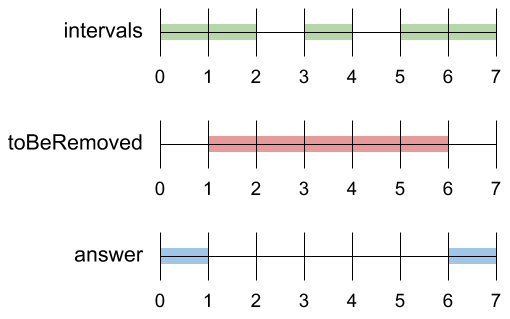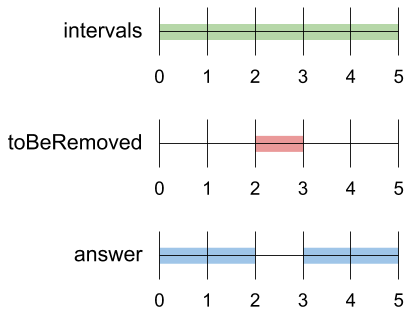1272. Remove Interval

A set of real numbers can be represented as the union of several disjoint intervals, where each interval is in the form `[a, b)`. A real number `x` is in the set if one of its intervals `[a, b)` contains `x` (i.e. `a <= x < b`).

You are given a sorted list of disjoint intervals `intervals` representing a set of real numbers as described above, where `intervals[i] = [ai, bi]` represents the interval `[ai, bi)`. You are also given another interval `toBeRemoved`.

Return the set of real numbers with the interval `toBeRemoved` removed from `intervals`. In other words, return the set of real numbers such that every `x` in the set is in `intervals` but not in `toBeRemoved`. Your answer should be a sorted list of disjoint intervals as described above.

Example 1:```Input: intervals = [[0,2],[3,4],[5,7]], toBeRemoved = [1,6]
Output: [[0,1],[6,7]]
```

Example 2:```Input: intervals = [[0,5]], toBeRemoved = [2,3]
Output: [[0,2],[3,5]]
```

Example 3:

```Input: intervals = [[-5,-4],[-3,-2],[1,2],[3,5],[8,9]], toBeRemoved = [-1,4]
Output: [[-5,-4],[-3,-2],[4,5],[8,9]]
```

Constraints:

• `1 <= intervals.length <= 104`
• `-109 <= ai < bi <= 109`

1272. Remove Interval
``````struct Solution;

impl Solution {
fn remove_interval(intervals: Vec<Vec<i32>>, to_be_removed: Vec<i32>) -> Vec<Vec<i32>> {
let l = to_be_removed;
let r = to_be_removed;
let mut res = vec![];
for interval in intervals {
if interval < l || interval > r {
res.push(interval);
} else {
if interval < l {
res.push(vec![interval, l.min(interval)]);
}
if interval > r {
res.push(vec![r.max(interval), interval]);
}
}
}
res
}
}

#[test]
fn test() {
let intervals = vec_vec_i32![[0, 2], [3, 4], [5, 7]];
let to_be_removed = vec![1, 6];
let res = vec_vec_i32![[0, 1], [6, 7]];
assert_eq!(Solution::remove_interval(intervals, to_be_removed), res);
let intervals = vec_vec_i32![[0, 5]];
let to_be_removed = vec![2, 3];
let res = vec_vec_i32![[0, 2], [3, 5]];
assert_eq!(Solution::remove_interval(intervals, to_be_removed), res);
}
``````